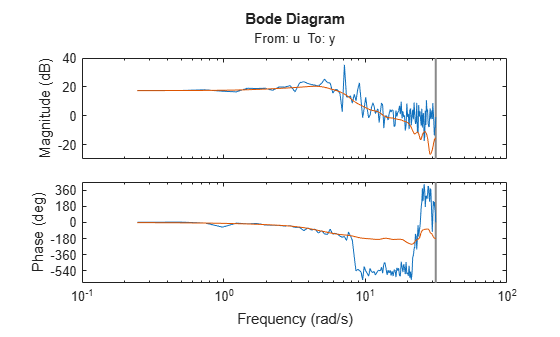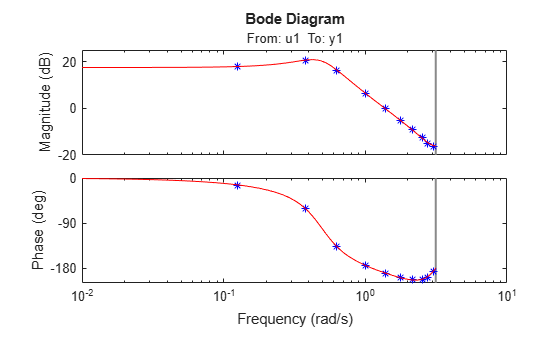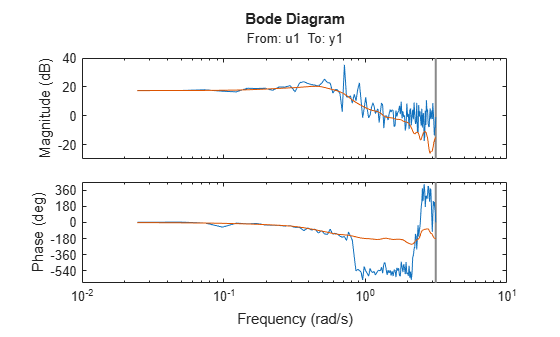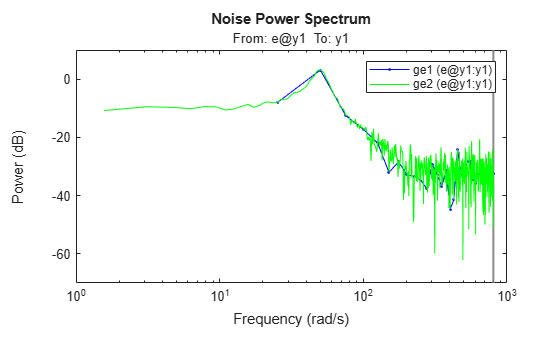# etfe

Estimate empirical transfer functions and periodograms

## Syntax

``g = etfe(data)``
``g = etfe(data,M)``
``g = etfe(data,M,N)``
``g = etfe(___,Name,Value)``

## Description

example

````g = etfe(data)` estimates a transfer function of the form: $y\left(t\right)=G\left(q\right)u\left(t\right)+v\left(t\right)$`data` contains time-domain or frequency-domain input/output data or time series data. `data` can be in the form of a `timetable`, comma-separated pair of numeric matrices, or time-domain or frequency-domain `iddata` object. If `data` contains time-domain input/output signals, then `g` is the ratio of the output Fourier transform to the input Fourier transform for the data.For nonperiodic data, the transfer function is estimated at 128 equally-spaced frequencies `[1:128]/128*pi/Ts`.For periodic data that contains a whole number of periods (`data.Period = integer` ), the response is computed at the frequencies `k*2*pi/period` for ```k = 0``` up to the Nyquist frequency.If `data` contains frequency-domain input/output signals, `g` is the ratio of output to input at all frequencies, where the input is nonzero.If `data` contains time-series data (no input channels), `g` is the periodogram, that is, the normed absolute square of the Fourier transform, of the data. The corresponding spectral estimate is normalized, as described in Spectrum Normalization and differs from the `spectrum` normalization in the Signal Processing Toolbox™ product.If `data` is a timetable, the software interprets the last variable is the single output variable. To change this interpretation, use the `InputName` and `OutputName` name-value arguments.```

example

````g = etfe(data,M)` applies a smoothing operation on the raw spectral estimates using a Hamming Window that yields a frequency resolution of about `pi/M`. The effect of `M` is similar to the effect of `M` in `spa`. `M` is ignored for periodic data. Use this syntax as an alternative to `spa` for narrowband spectra and systems that require large values of `M`. ```

example

````g = etfe(data,M,N)` specifies the frequency spacing `N` for nonperiodic data.For nonperiodic time-domain data, `N` specifies the frequency grid `[1:N]/N*pi/Ts` rad/`TimeUnit`. When not specified, `N` is 128.For periodic time-domain data, `N` is ignored.For frequency-domain data, the `N` is `fmin:delta_f:fmax`, where ```[fmin fmax]``` is the range of frequencies in `data`, and `delta_f` is `(fmax-fmin)/(N-1)` rad/`TimeUnit`. When not specified, the response is computed at the frequencies contained in data where input is nonzero.```
````g = etfe(___,Name,Value)` uses additional model options specified by one or more name-value arguments. For example, specify the input and output signal variable names using ```g = etfe(data,'InputName',["u1","u3"],'OutputName',["y1","y4"])```. You can use this syntax with any of the previous input-argument combinations.```

## Examples

collapse all

`load sdata1 tt1;`

Estimate empirical transfer function and smoothed spectral estimate.

```ge = etfe(tt1); gs = spa(tt1);```

Compare the two models on a Bode plot.

`bode(ge,gs)`Generate a periodic input, simulate a system with it, and compare the frequency response of the estimated model with the original system at the excited frequency points.

Generate a periodic input signal and output signal using simulation.

```m = idpoly([1 -1.5 0.7],[0 1 0.5]); u = iddata([],idinput([50,1,10],'sine')); u.Period = 50; y = sim(m,u);```

Estimate an empirical transfer function.

`me = etfe([y u]);`

Compare the empirical transfer function with the original model.

`bode(me,'b*',m,'r')`Perform a smoothing operation on raw spectral estimates using a Hamming Window and compare the responses.

`load sdata1 umat1 ymat1`

Estimate empirical transfer functions with and without the smoothing operation.

```ge1 = etfe(umat1,ymat1); ge2 = etfe(umat1,ymat1,32);```

Compare the models on a Bode plot.

`ge2` is smoother than `ge1` because of the effect of the smoothing operation.

`bode(ge1,ge2)`Estimate empirical transfer functions with low- and high-frequency spacings and compare the responses.

`load iddata9`

Estimate empirical transfer functions with low and high frequency spacings.

```ge1 = etfe(z9,[],32); ge2 = etfe(z9,[],512);```

Plot the output power spectrum of the two models.

`spectrum(ge1,'b.-',ge2,'g')`## Input Arguments

collapse all

Uniformly sampled estimation data, specified as a timetable, comma-separated matrix pair, time-domain or frequency-domain `iddata` object, cell array of timetables, or cell array pair of matrices, as the following sections describe.

#### Timetable

Specify `data` as a `timetable` that uses a regularly spaced time vector. `data` contains variables representing input and output channels.

For multiexperiment data, specify data as an Ne-by-1 cell array of timetables, where Ne is the number of experiments. The sample times of all the experiments must match.

When you use timetables for estimation, you can use all the variables or specify a subset of channels to use. To select individual input and output channels to use for estimation, use the `InputName` and `OutputName` name-value arguments.

For time series data, either specify `data` as a single-variable timetable containing only an output variable, or use `InputName` and `OutputName` name-value arguments to specify only output variables for estimation.

For example, `sys = etfe(data,'OutputName',"y2",'InputName',[])` estimates the time series model `sys` from the timetable `data` using the output variable `y2` and no input variables.

#### Comma-Separated Matrix Pair

Specify `data` as a comma-separated pair of matrices u,y that contain uniformly sampled input and output time-domain signal values. Matrix-based data provides no sample-time information. The software assumes that the sample time is one second. Using matrix-based data for continuous-time systems is not recommended.

For SISO systems, specify u,y as column vectors with a length of Ns, where Ns is the number of samples.

For MIMO systems, specify u,y as a matrix pair with the following dimensions:

• uNs-by-Nu, where Nu is the number of inputs

• yNs-by-Ny, where Ny is the number of outputs

For multiexperiment data, specify u,y as a pair of 1-by-Ne cell arrays, where Ne is the number of experiments.

For time series systems, specify an empty u, that is, `[]`,y.

#### Data Object

An estimation data object, specified as a time-domain or frequency-domain `iddata` object that contains uniformly sampled input and output values. The data object can have one or more output channels and zero or more input channels. By default, the software sets the sample time of the model to the sample time of the estimation data.

For more information about working with estimation data types, see Data Domains and Data Types in System Identification Toolbox.

Frequency resolution, specified as a positive scalar.

For more information about working with estimation data types, see Data Domains and Data Types in System Identification Toolbox.

Frequency spacing, specified as a positive scalar. For frequency-domain data, the default frequency spacing is the spacing inherent in the estimation data.

### Name-Value Arguments

Specify optional pairs of arguments as `Name1=Value1,...,NameN=ValueN`, where `Name` is the argument name and `Value` is the corresponding value. Name-value arguments must appear after other arguments, but the order of the pairs does not matter.

Before R2021a, use commas to separate each name and value, and enclose `Name` in quotes.

Example: `sys = etfe(data,'InputName',"u2")`

Input channel names, specified as a string, character vector, string array, or cell array of character vectors.

If you are using a timetable for the data source, the names in `InputName` must be a subset of the timetable variables.

Example: `sys = etfe(tt,__,'InputName',["u1" "u2"])` selects the variables `u1` and `u2` as the input channels from the timetable `tt` to use for the estimation.

Output channel names, specified as a string, character vector, string array, or cell array of character vectors.

If you are using a timetable for the data source, the names in `OutputName` must be a subset of the timetable variables.

Example: `sys = etfe(tt,__,'OutputName',["y1" "y3"])` selects the variables `y1` and `y3` as the output channels from the timetable `tt` to use for the estimation.

Sample time, specified as the comma-separated pair consisting of `'Ts'` and the sample time in the units specified by `TimeUnit`. When you use matrix-based data (`u`,`y`), you must specify `Ts` if you require a sample time other than the assumed sample time of 1 second.

To obtain the data sample time for a timetable `tt`, use the timetable property `tt.Properties.Timestep`.

Example: `etfe(umat1,ymat1,___,'Ts',0.08)` computes a model with sample time of 0.08 seconds.

## Output Arguments

collapse all

Transfer function estimate, returned as an `idfrd` model.

Information about the estimation results and options used is stored in the model's `Report` property. `Report` has the following fields:

Report FieldDescription
`Status`

Summary of the model status, which indicates whether the model was created by construction or obtained by estimation.

`Method`

Estimation command used.

`WindowSize`

Size of the Hamming window.

`DataUsed`

Attributes of the data used for estimation, returned as a structure with the following fields.

FieldDescription
`Name`

Name of the data set.

`Type`

Data type.

`Length`

Number of data samples.

`Ts`

Sample time.

`InterSample`

Input intersample behavior, returned as one of the following values:

• `'zoh'` — Zero-order hold maintains a piecewise-constant input signal between samples.

• `'foh'` — First-order hold maintains a piecewise-linear input signal between samples.

• `'bl'` — Band-limited behavior specifies that the continuous-time input signal has zero power above the Nyquist frequency.

`InputOffset`

Offset removed from time-domain input data during estimation. For nonlinear models, it is `[]`.

`OutputOffset`

Offset removed from time-domain output data during estimation. For nonlinear models, it is `[]`.

For more information on using `Report`, see Estimation Report.

## Version History

Introduced before R2006a

expand all